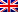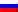# Logic programming

Contenus

## Logic programming

Logic programming is the declaration of a series of facts and rules of deduction. The execution of a program consists in proving a theorem. The program answers whether or not the theorem can be proved from the statements made beforehand.

Logic programming is different from procedural programming (C, JAVA, etc.), the calculations are done in the form of logical proofs. We speak of declarative programming: the program describes a situation corresponding to a problem to be solved. Declarative programming is not incompatible with object structuring.

The formulas expressed in Prolog are restricted to Horn clauses and first order logic. A Horn clause is a clause with at most one positive literal. These will not be explained but implicit in the explanations of the Prolog language.

# Rules, facts and goals

If the Horn clause has one positive literal and at least one negative literal, they are rules :
qqbe x, y, z, ¬child (x, y) ∨ ¬child (y, z) ∨ grand_child (x, z) which equals
child (x, y) ∧ child (y, z) ⇒ grand_child (x, z)

is written in Prolog: grandchild_of (X, Z): - child_of (X, Y), child_of (Y, Z)

You can write Horn clauses without a negative literal (positive Horn clauses), but with only one positive literal. Those are facts : child_of ('Bernard', 'Bernadette').

We can write Horn clauses without positive literals, (negative Horn clauses), but with only negative literals. Those are goals : ? -little_child_of (B, 'germaine'), nephew (X, Y).

A program is therefore a set of facts and rules, all in the form of Horn clauses. The Prolog interpreter uses this knowledge base to meet goals.

# Prolog syntax

The syntax consists of:

• Constants : integer or floating point numbers, booleans, identifiers and strings.
• Variables : identified by character strings starting with a capital letter or an underscore (if unknown, ie of no interest to the user). Variable is in the mathematical sense, it always represents the same object and does not change value in the same branch of proof.
• Predicate : identified by character strings starting with a lowercase letter. A predicate has an arity (the number of arguments it requires). Parameters are separated by a comma, equivalent to AND; the OR is a semicolon.

Rules and facts:

• a fact is a predicate with constants as parameters: parent ('Bernard', 'Bernardo')
• a rule consists of a head (positive literal) and a tail (conjunction of negative literals ending with a period: nephew (X, Y): - son (X, Z), brother_or_sister (Y, Z).

# Write a Prolog program

A program is a list of facts and rules. We group together the rules which have the same leading predicate and the facts which relate to the same predicate. The order in which the rules are declared can have an impact on the goals.

`child_of ('roger', 'gertrude'). child_of ('gertrude', 'germaine'). child_of ('marcel', 'robert'). brother_or_sister ('gertrude', 'robert'). nephew (X, Y): - son (X, Z), brother_or_sister (Y, Z). grand_child_of (X, Y): - child_of (X, Z), child_of (Z, Y).`

A constant ('roger') covers the whole program while a variable only exists in the clause where it is found. So the variable X of nephew (X, Y) is not the same as that of grand_child_ of (X, Y). On the other hand, it is the same as in the conjunction which is associated with it.

Once the programs loaded and thus the base of facts and rules constituted, it is possible to ask questions. This is called a demonstration or a question resolution. A question arises by replacing in a rule or making a constant or variable by a '?'. To find out all the rules of a type, write the name of the rule containing variables followed by a '?' : human (H)? is used to know all the humans of the base.FRFRENRUES